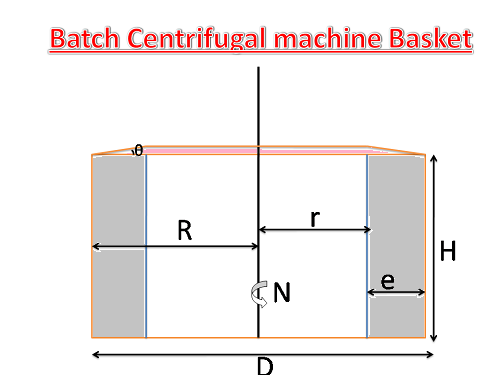# Batch Centrifugal Machine Capacity and Gravity Factor Calculation

## Formulas for Batch Centrifugal Machine Capacity and Gravity Factor with Online Calculator

The batch centrifugal machines have been using to handle white and refined sugar massecuites as well as for affination of raw sugar in the sugar refineries.

The batch machines were fully satisfying the sugar industry requirements for high sugar quality such as: low color, no lumps and low residual moisture content.

### Parameters to be required for calculation:Basket Shell Internal Diameter in meters = D

Thickness of the massecuite in meters   = e (Generally “e” value taken 0.14D for theoretical and 0.12D  for practical)

Basket top plate angle to horizontal level in Deg.= θ (Generally this value having 5deg.)

Height of the basket in meters= H

RPM of the basket (maximum RPM in Cycle)  = N

Time required for one cycle in sec    = T

Density of the massecuite in gm/ml    = S

### Formulas for Calculation :

Volume of the massecuite per cycle in M3  (M3/cycle)  = V = π e[ H(D-e) + e tanθ ((3D-4e)/6)]

No. of cycles per hour  (cycle/hr) =C =3600/T

Capacity of the machine (Theoretically) (Q)

Q = C x V in M3/hr  (M3/cycle x Cycle/hr)

=Q x S in tons/hr

But in practically calculating the capacity of machine takes the following considerations.

1. Take 1 to 2 empty cycles during in an hour (Depends on massecuite condition) so consider one empty full cycle in total cycles per hour.

2. While charging the massecuite is always purged, spelling out some molasses such that creating space for further massecuite. This is called purging capacity. It is 10% more than theoretical capacity.

Capacity of the machine (Practically)  =Q

[(C-1) x V] x 1.1 in M3/hr

Q x S in tons/hr

Mean Equivalent Radius in mtrs = Rm = 2/3 [(R3 – r3) / (R2 – r2)]

Gravity factor     = G   = Rm x 1119 x (N / 1000)2

Gravity factor (General formula)   = G   = D x N2 / 1800

##### Explanation for gravity factor formula

Gravity Factor   =     Centrifugal force /  Gravitational force

=   m x  Rm x w2   / m x g.

Where Rm is M.E.R. and

w = angular velocity   = 2 π N  /60      g = 9.8 m/sec2

Gravity Factor =[ Rm . X  1 X (2πN / 60 )2 ] / 9.8

= Rm x 1119 x (N / 1000)2

#### Online Calculator to find Batch Centrifugal Machine Capacity and Gravity Factor

Some Related articles:

Phosphoric Acid(H3PO4) in sugar process industry and online calculator.

Definitions in Steam Properties and Online Steam Table

Sugar industry Job vacancies Information | Job Vacancies in Sugar Industry.

Sugar Industry Related Important Websites | sugar Technology.

Sugar Tech | Sugar Technology related articles .

Sugar Industry Equipment Design and Drawing Online Calculations.

Thumb Rules for Sugar factory Equipment Design Sizing.

Hi friends Thanks for reading. I Hope you liked it.  Give feed back, comments and please don’t forget to share it

## 14 thoughts on “Batch Centrifugal Machine Capacity and Gravity Factor Calculation”#### Awanish kumar

(May 8, 2017 - 9:21 am)

How you are converting that volume into kg/charge
Volume of the massecuite per cycle in M3 = = π e[ H(D-e) + e tanθ ((3D-4e)/6)]

No. of cycles per hour =C =3600/T

Capacity of the machine (Theoretically) = Q = C x V in M3/hr
=Q x S in tons/hr

& Gravity factor = G = Rm x 1119 x (N / 1000)2

Is not coming accordingly.#### siva alluri

(May 9, 2017 - 2:05 pm)

Thank you for your commenting sir
Explanation for above given default example in calculator

 Practical capacity Theoretical capacity Tanθ 0.087 0.087 H(D-e) 1.586 1.550 (3D-4e)/6) 0.714 0.691 e tanθ ((3D-4e)/6) 0.013 0.014 π e[ H(D-e) + e tanθ ((3D-4e)/6)] 1.024 1.169 M3 /cycle V x Density x 1000 x1.1(10%extra for pratical capacity only)) 1644.443 1706.586 Kgs/Cycle Capacity of the machine x No. of cycles per hour 31244.42 34131.72 Kgs/hr 31.24 34.13 Ton/hr R = D/2 0.850 0.850 r = R-e 0.646 0.612 Rm = 2/3 [(R3 – r3) / (R2 – r2)] 0.753 0.737 Rm x 1119 x ( N / 1000)2 928.526 910.000#### NPSINGH

(November 13, 2017 - 12:47 pm)

NICE#### Nausheen

(December 15, 2017 - 9:00 am)

Hello sir,
Do you have the data and calculations for continuous centrifugals and those for the drying section?#### siva alluri

(December 15, 2017 - 4:02 pm)

we published soon regarding this top#### Ara

(December 18, 2017 - 7:55 am)

Hello Sir,
your calculation is very helpful. Since i have study about the centrifugal for the sugar processing, i need to explain about the calculation in details. I have try using your calculation and i got the answer, but when i calculate manually, it is too different. Could you help me ?#### ARUN KUMAR PAL

(February 9, 2018 - 7:52 am)

very good ormula.
thanks.#### B. N Amarendra

(March 31, 2018 - 1:36 pm)

sir, Pl tell me about massecuite reheater design calculation#### siva alluri

(April 2, 2018 - 4:41 am)

We Will publish soon(July 7, 2018 - 9:05 am)

HOW TO CALCULATE CENTRIFUGAL MACHINE SCREEN OPENING#### siva alluri

(July 12, 2018 - 1:43 pm)

It is very simple
1. Find the hole dia of the screen – i.e mesh size ex: 0.06 (60 micron) or 0.09 ( 90 micron)
2. Find the no of holes per sq.cm.
3. Find the perforated area of the each segment of the screen and calculate total area in sq.cm
Now we can find the screen opening area#### Santosh Kumar Bakkoli

(September 23, 2018 - 4:10 am)

Sir I m centrifugal mate batch machine Conti machine parts name(February 18, 2019 - 1:21 pm)

Nice. Information
I have a dout sir,
Can you tell me how calculate blower capacity for centrifugal machine ??#### siva alluri

(February 20, 2019 - 2:55 pm)

Ok we will provide soon